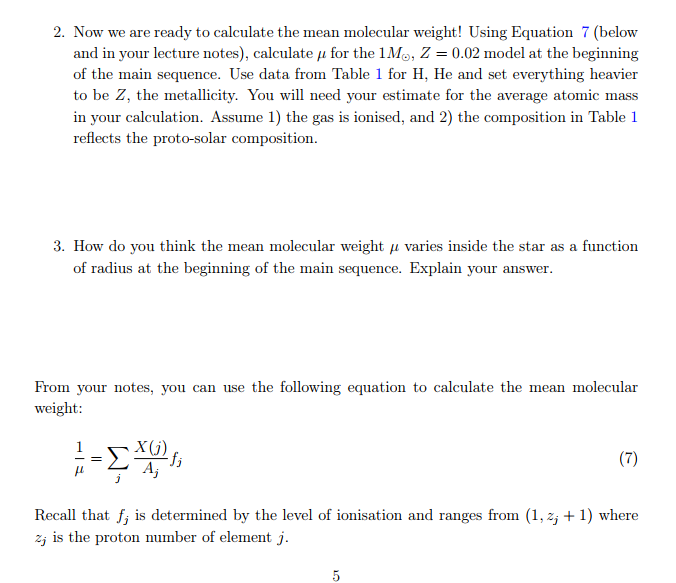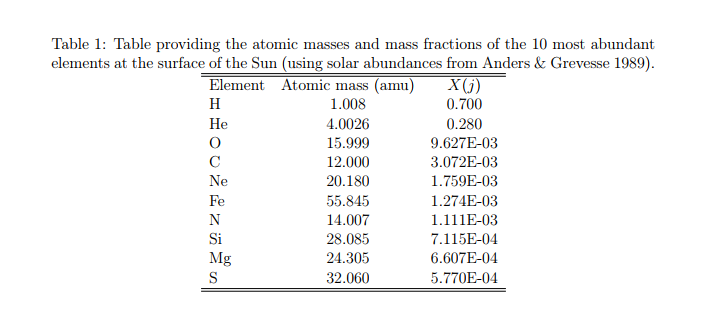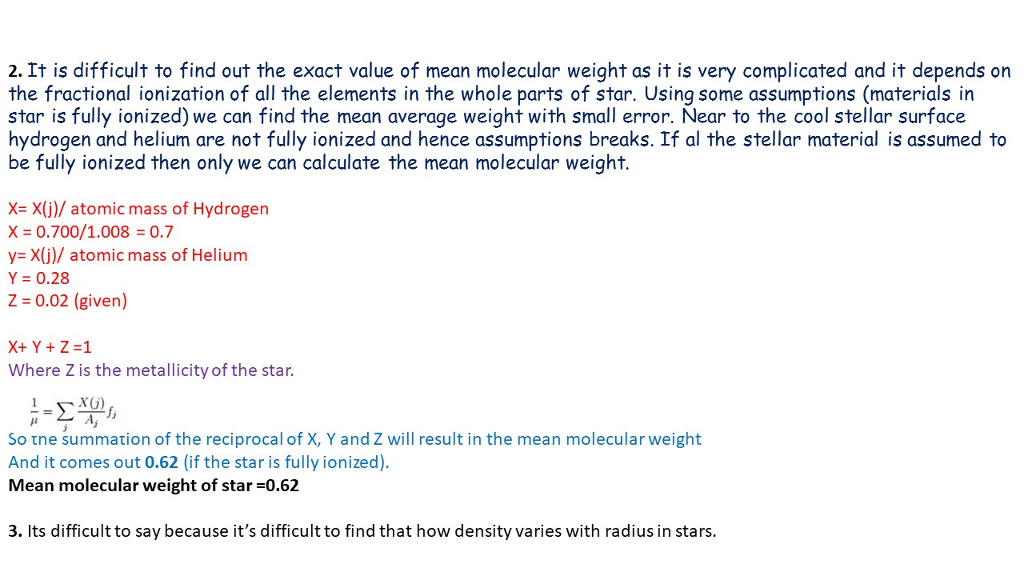# Homework Solution: Now we are ready to calculate the mean molecular weight! Using Equation 7 (below and in your…Now we are ready to calculate the mean molecular weight! Using Equation 7 (below and in your lecture notes), calculate mu for the 1M , Z = 0.02 model at the beginning of the main sequence. Use data from Table 1 for H, He and set everything heavier to be Z, the metallicity. You will need your estimate for the average atomic mass in your calculation. Assume 1) the gas is ionised, and 2) the composition in Table 1 reflects the proto-solar composition. How do you think the mean molecular weight mu varies inside the star as a function of radius at the beginning of the main sequence. Explain your answer. From your notes, you can use the following equation to calculate the mean molecular weight: 1/mu = sigma X (j)/A_j f_j Recall that f_j is determined by the level of ionisation and ranges from (1, z_j + 1) where z_j is the proton number of element j.